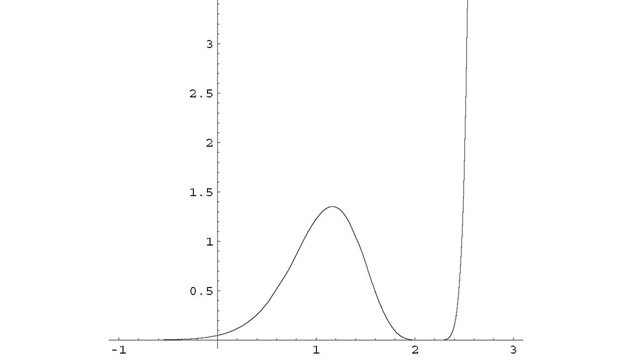abstractmath.org

help with abstract math

`Produced by Charles Wells.  Home.   Website Contents     Website Index  `
`Back to Useful Behaviors head `

Posted 5 February 2009

DOING MATH: Zooming and chunking

Here is an example of how someone with some practice at dealing with abstract math will analyze a problem.  This example requires only first year calculus.

# A function

Suppose your research has come up with the functiondefined for real numbers x.  You want to understand how this function behaves.  If I were looking at it, I would go through some process like this:

1)     Is f(x) defined everywhere? (Meaning, is the denominator never  0?)  Yes,is positive for all real numbers x, sohas to be positive.2)     In fact, I just noticed thatmust always be greater than 1.  This means that f(x) is always less than.3)     f(x) is a positive number times a 6th power, so it is always nonnegative.

4)     Could f(x) be zero?  Yes, the numerator is zero when, and that is the only place.  (Every real number has exactly one real cube root.)5)     When x is positive,is extremely close to 1, so when you get very far out on the x axis, f(x) looks just like.  This means it gets big very quickly as you go to the right.6)     …Well, big except near(about 2.1544), where it has to be zero.  So it must dip down to 0 at 2.1544 and that start going up again very fast.7)     That means it has to have a local minimum at.  Ye gods, I don’t want to take that derivative… (If I did take the derivative I would have found out that f(x) has a local maximum between 1 and 2).8)     What about negative x?  Well, thereis very large and grows a heck of a lot faster than any polynomial, so the graph to the left of the y-axis ought to hug the x-axis (while staying just above it).…and so on.  It is worth taking a very close look at what goes on in a mathematician’s head when they go through a process of analysis like this. What happens with me is that I zoom in and out and when I have zoomed out I see things in chunks:

I look at the big picture

then zoom in on some detail that tells me something about the graph

then zoom out and look at the big picture again

then zoom in to another detail…

When I am looking at the big picture

I don’t see all the details

I just see chunks of formula with some relevant properties

### Some Details

Let’s look at the first few steps in detail.  In each step, I am selective in what details I pay attention to and lump the stuff I am not paying attention to into “something”, perhaps with a qualification such as “something > 0”.   The lumping part is called chunking or encapsulation in the math ed literature.  In the steps just above, the chunks are in red and the relevant detail is in blue.

1)so it is always defined.2)so.3), which is, so it is always nonnegative.4)  Every real number has exactly one cube root, sowhenand nowhere else.  Sinceand the only time a fraction is zero when its numerator is zero,is the only x for which f(x) = 0.And so on…

#### People are different

Not every mathematician would do this in exactly the way I did.

¨  Many would have lumped steps (1) and (2) into one step, noticing right away that the denominator was not only positive but greater than 1.  I wrote the steps the way I did because that was in fact the order in which I noticed things.

¨  I could have referred to .0002 repeatedly as “something positive”.  In fact when I was going through the process I always thought of it as .0002, so I wrote it that way.  You might do it differently.

¨  In step (3) I referred to the fact that.  I could have noticed that, but that is irrelevant. I did not try to remember more detail than I had to.I have described a way of understanding something complicated by focusing on one detail and lumping all the other stuff into “something”, or “something with some property”.  On this website I will call it the zoom and chunk method.

Experts characteristically use the zoom and chunk method

to understand something complex.

You should too.

### The graphMost mathematicans faced with a function like this would graph it using Mathematica or some other program that does math.  (The graph above was done by Mathematica.)  That is a flaw with this example, since it ignores actual practice.  I wanted an example that would be accessible to people getting into abstract math, and this one requires only first year calculus.  More abstract and advanced problems might be less amenable to a computer math program.

### The graph tells less than you think

In any case, the graph does not show you some aspects of the graph that the analysis above does show.  You can’t tell just from looking at the graph that the function is asymptotic to 0 in the negative direction and increases rapidly to the right of.  (It appears to do these things but you could be fooled.)  You can’t tell it is zero only at one place, either.### Acknowledgments

Thanks to Olaf Stackleberg for corrections.